6202

Chemistry Inorganic Chemistry Level: Misc Level

The two suffixes that indicate the presence of oxygen in a polyatomic ion are

a.-ite &- ide

b. - ite & -ide

c. -ate &- ide

d.- thio &- penta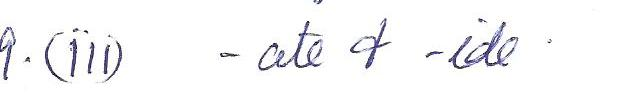6201

Chemistry Inorganic Chemistry Level: Misc Level

The lewis structure for methane, CH4, has

a. 2 double bonds

b. 1 triple bond

c. 4 single bonds

d. 2 single bonds & 2 double bonds6200

Chemistry Inorganic Chemistry Level: Misc Level

the correct formula for Magnesium Oxide is

a.MgO2

b.MgO

c Mg2O

d.Mg2O3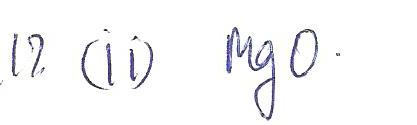6199

Chemistry Inorganic Chemistry Level: Misc Level

VSEPR theory staes that the repulsion between electron pairs surrounding an atom causes

a.these pairs to be as far apart as possible

b.an electron sea to form

c.a bond to break

d.a covalent bond to form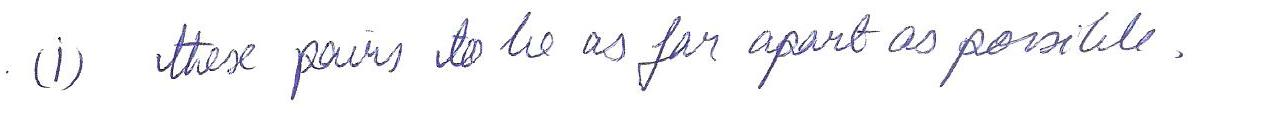6198

Chemistry Inorganic Chemistry Level: Misc Level

the charge of an ion is

a. always positive

b. always negative

c.either positive or negative

d.zero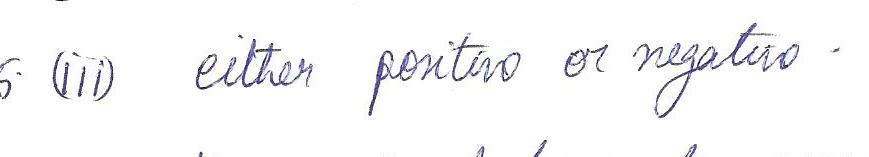6197

Chemistry Inorganic Chemistry Level: Misc Level

The tendency of atoms to gain or lose electrons so that their ouer orbitals are filled with electrons is the

a.vseper theory

b. Quartet rule

c.Octet Rule

dElectronegativity rule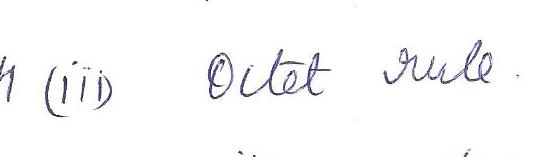6196

Chemistry Inorganic Chemistry Level: Misc Level

Compounds that are electrically neutral must have equal numbers of

a.anions &cations

b.positive & negative charges

c.moleecules

d.ionic bond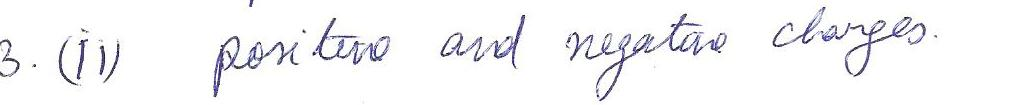6195

Chemistry Inorganic Chemistry Level: Misc Level

A covalent bond in which two atoms share three pairs of electrons between is called a (n)

a. single bond

b. double bond

c.triple bond

d.metallic bond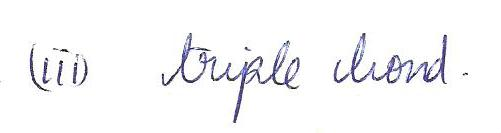6194

Chemistry Inorganic Chemistry Level: Misc Level

A polar covalent bond is likely to form between two atoms that

a.differ in electronegativity

b. are of simliar size

c. differ in state of matter

d. have the same number of electrons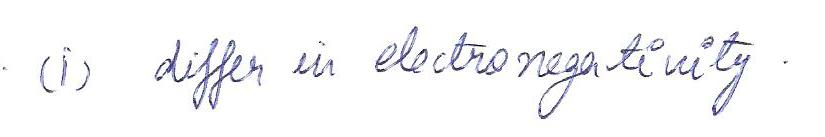6193

Chemistry Inorganic Chemistry Level: Misc Level

an ion that has a similar electron configuration to that of neon is

a.CI-

b. Na

C. Na+

d.O_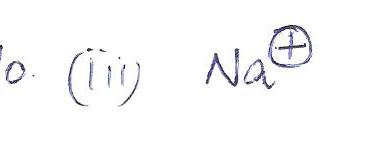6192

Chemistry Inorganic Chemistry Level: Misc Level

According to the octet, a calcium atom has a tendency to

a.lose one electron

b.lose two electrons

c.gain one electron

d.gain two electrons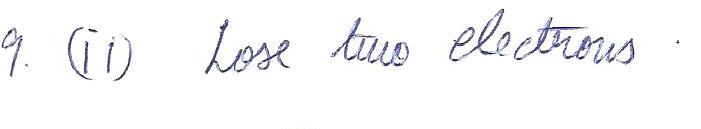6191

Chemistry Inorganic Chemistry Level: Misc Level

The correct Lewis structure for the boron atom has

a.one single valence electron

b.two single valence electron

c.three single valence electrons

d. one pair of electrons and 3 single valence electron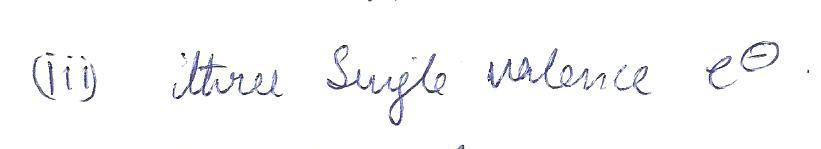6190

Chemistry Inorganic Chemistry Level: Misc Level

lonic bonding occurs between

a.ions of opposite electrical charge

b.ions of the same electrical charge

c. ions of the same size

d. ions of the same size and opposite charge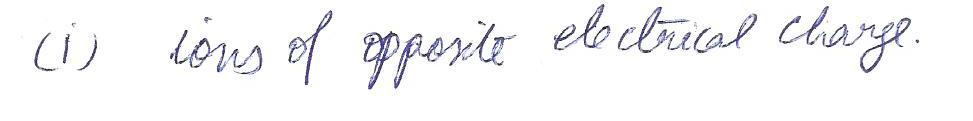6189

Chemistry Inorganic Chemistry Level: Misc Level

The correct name for the salt with the formula CaI2 is

a. calcium iodie

b.calcium di-iodine

c. calcium iodine

d. dicalcium iodide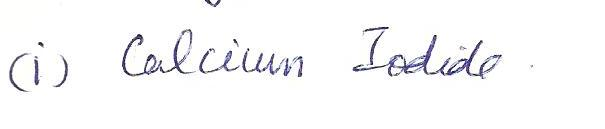6188

Chemistry Inorganic Chemistry Level: Misc Level

Multiple covalent bonds may be found in the atoms that contain carbon, nitrogen, or

a. helium

b. argon

c. hydrogen

d. oxygen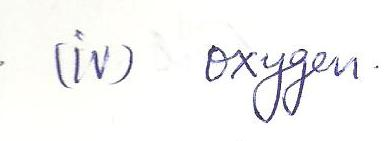Displaying 31-45 of 61 results.